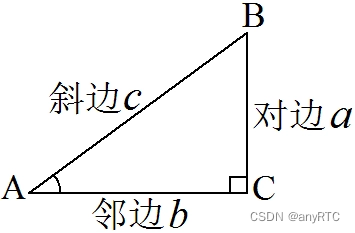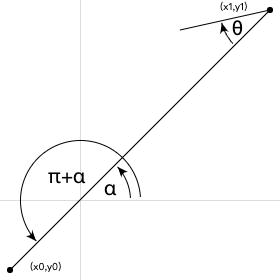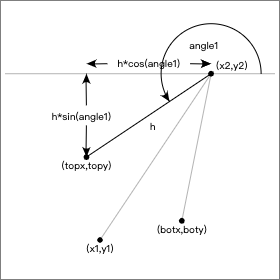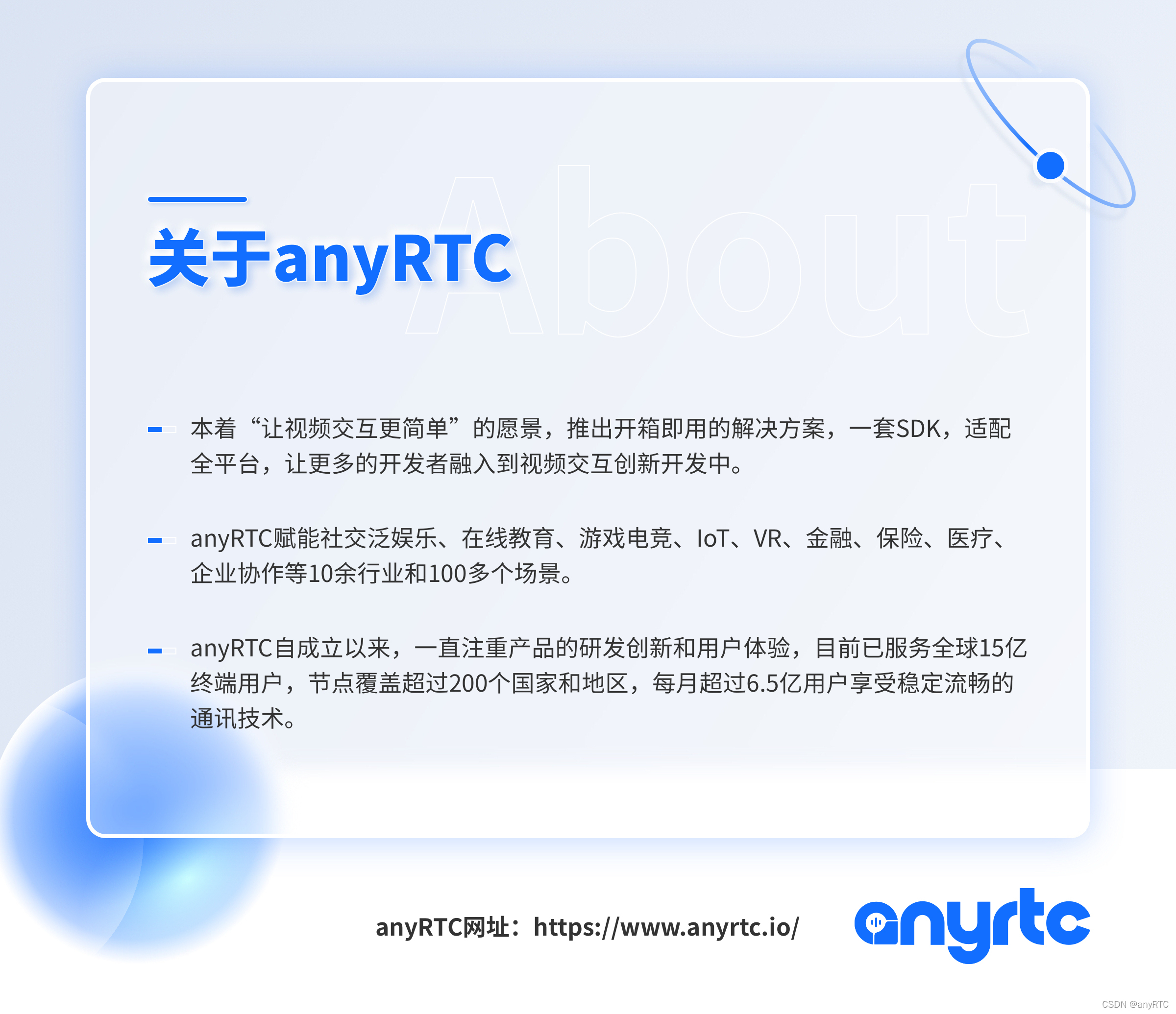# web 技术分享| 白板 SDK 之函数和方程式的运用

• 2022 年 1 月 19 日
• 本文字数：3117 字

阅读完需：约 10 分钟- 框选工具是否框中图形，选中哪些图形?- 鼠标是否选中图形（空心形状还是填充形状）？- 图形是否相交/切？- 等等

• 复习一下：什么是直角坐标系、三角函数、勾股定理、圆的标准方程式

• 这些函数以及方程式都应用在哪些场景？

• 实战练习

## 直接坐标系## 勾股定理• 计算两个坐标点之间的距离

• 计算两形状是否相交（切）

• 判断坐标是否在圆上

• 高阶：计算物理移动的速度

## 三角函数

• 已知两条边，求两条边的夹角

• 已知一条边和这条边的夹角，求夹角的另一条边

• 绘制箭头

• 计算旋转角度

## 实战练习

• 计算两个图形是否相交（切）

• 绘制箭头

• 笔锋（签字笔、钢笔、毛笔）

### 计算两个图形是否相交（切）

• 圆形只需要判断两个圆心之间的距离是否小于两圆半径之和

•• 矩形则需要判断两个矩形中心点的：

• x 轴的距离是否小于两矩形宽度之和的 1 / 2

• y 轴的距离是否小于两个矩形高度之和的 1 / 2

### 绘制箭头

• 第一步，我们需要绘制一条直线，从 A(x0, y0) 点到 B(x1, y1) 点

• 第二步，设置箭头的长度 headlen 和箭头与之前的夹角 θ

• 第三步，计算这条线与 X 轴正方向的夹角// 将弧度制转换成度数  const angle = Math.atan2(y1 - y0, x1 - x0) * 180 / Math.PI;

• 第四步，推理出箭头左右（上下）两侧斜线与 X 轴正方向的夹角

• 根据步骤三我们可以算出箭头两侧的斜线与 x 轴正方向之间的平面角度(弧度值)，已知箭头与直线的夹角 θ 是固定的，另外已知直线与 X 轴正方向的夹角，只需要减去或者加上θ 就是箭头两侧的斜线与 x 轴正方向之间的平面角度。

• 大家可以在箭头末端作出延伸线（如下图），箭头上边（下图左边）的夹角为 π + α - θ，箭头下边（图右边）的夹角为 π + α + θ// 箭头的左侧夹角  const angle1 = (angle - theta) * Math.PI / 180;  // 箭头的右侧夹角  const angle2 = (angle + theta) * Math.PI / 180;  // 计算出右侧箭头的坐标点  const topX = headlen * Math.cos(angle1);  const topY = headlen * Math.sin(angle1);  // 计算出左侧箭头的坐标点  const botX = headlen * Math.cos(angle2);  const botY = headlen * Math.sin(angle2);

### 笔锋

• 落点是个圆形

• 通过计算画笔移动的距离和时间得到画笔的速度，通过速度的快慢来设置画笔的粗细

• 使用二次贝塞尔曲线或者三次贝塞尔曲线进行绘制

• 收笔画笔粗细慢慢变细（可以根据笔的类型：钢笔、毛笔、签字笔等自由发挥）

• 第一步，通过勾股定理计算出 AB 两点之间的距离

  // 计算两点 X 轴上的距离  const x = x1 - x0;  // 计算两点 Y 轴上的距离  const y = y1 - y0;  // 计算两点的直线距离  const s = Math.sqrt(Math.pow(x, 2) + Math.pow(y, 2));

• 第二步，通过速度计算公式计算出两点之前移动的速度

• 一般白板中我们会记录每个坐标绘制的时间，因此我们可以很轻易的计算出 A 到 B 的耗时 t，套用速度计算公式，我们就可以计算出，A 到 B 绘制的速度 v

  // 速度计算公式  速度 v = 距离 s / 时间 t

• 第三步，根据速度快慢来调整画笔的粗细

• 最后我们根据速度的快慢，然后根据可变的速度比例，计算出速度在多少以下变粗，多少以下变细。

### 其他

• 图形旋转 - 使用矩阵转换的公式

• 动画 - 抛物线、匀速运动，加速运动，重力加速

• 等等

## 参考文献## 评论